##### Calculus Essentials For Dummies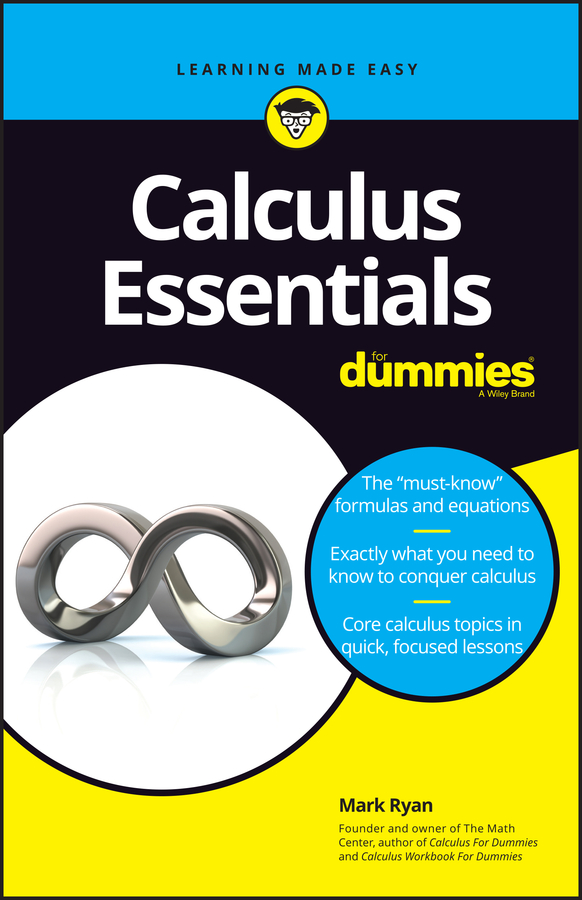Every time you get in your car, you witness differentiation firsthand. Your speed is the first derivative of your position. And when you step on the accelerator or the brake — accelerating or decelerating — you experience a second derivative.

If a function gives the position of something as a function of time, the first derivative gives its velocity, and the second derivative gives its acceleration. So, you differentiate position to get velocity, and you differentiate velocity to get acceleration.

Here’s an example. A yo-yo moves straight up and down. Its height above the ground, as a function of time, is given by the function, where t is in seconds and H(t) is in inches. At t = 0, it’s 30 inches above the ground, and after 4 seconds, it’s at height of 18 inches.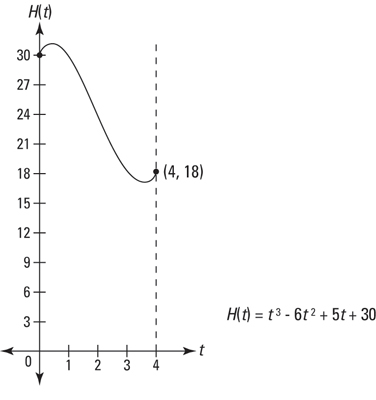Figure 1

The yo-yo’s height, from 0 to 4 seconds
Velocity, V(t) is the derivative of position (height, in this problem), and acceleration, A(t), is the derivative of velocity. Thus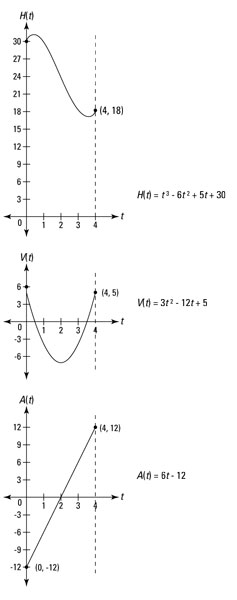Figure 2

The graphs show the yo-yo’s height, velocity, and acceleration functions from 0 to 4 seconds.

## Velocity versus speed

Your friends won’t complain — or even notice — if you use the words “velocity” and “speed” interchangeably, but your friendly mathematician will complain. Here’s the difference.

For the velocity function in Figure 2, upward motion is defined as a positive velocity and downward velocity is defined as a negative velocity — this is the standard way velocity s treated in most calculus and physics problems. (If the motion is horizontal, going right is a positive velocity and going left is a negative velocity.)

Speed, on the other hand, is always positive (or zero). If a car goes by at 50 mph, for instance, you say its speed is 50, and you mean positive 50, regardless of whether it's going to the right or the left.

For velocity, the direction matters; for speed it doesn’t. In everyday life, speed is a simpler idea than velocity because it agrees with common sense. But in calculus, speed is actually the trickier idea because it doesn’t fit nicely in the three-function scheme shown in Figure 2.

You need to keep the velocity-speed distinction in mind when analyzing velocity and acceleration. For example, if an object is going down (or to the left) faster and faster, its speed is increasing, but its velocity is decreasing because its velocity is becoming a bigger negative (and bigger negatives are smaller numbers). This seems weird, but that’s the way it works.

And here’s another strange thing: Acceleration is defined as the rate of change of velocity, not speed. So, if an object is slowing down while going in the downward direction, and thus has an increasing velocity — because the velocity is becoming a smaller negative — the object has a positive acceleration. In everyday English, you’d say the object is decelerating (slowing down), but in calculus class, you say that the object has a negative velocity and a positive acceleration.

By the way, “deceleration” isn’t exactly a technical term, so you should probably avoid it in calculus class. It’s best to use the following vocabulary: “positive acceleration,” “negative acceleration,” “speeding up,” and “slowing down.”

## Maximum and minimum height

Maximum and minimum height of H(t) occur at the local extrema you see in Figure 1. To locate them, set the derivative of H(t) — that’s V(t) — equal to zero and solve.

These two numbers are the zeros of V(t) and the t-coordinates — that’s time-coordinates — of the max and min of H(t), which you can see in Figure 2. In other words, these are the times when the yo-yo reaches its maximum and minimum heights. Plug these numbers into H(t) to obtain the heights:

H (0.47) ≈ 31.1

H (3.53) ≈ 16.9

So, the yo-yo gets as high as about 31.1 inches above the ground at t ≈ 0.47 seconds and as low as about 16.9 inches at t ≈ 3.53 seconds.

## Total displacement

is defined as the final position minus the initial position. So, because the yo-yo starts at a height of 30 and ends at a height of 18,

Total displacement = 18 – 30 = –12.

This is negative because the net movement is downward.

Average velocity is given by total displacement divided by elapsed time. Thus,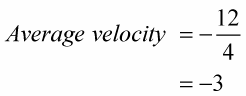This negative answer tells you that the yo-yo is, on average, going down 3 inches per second.

## Maximum and minimum velocity

Maximum and minimum velocity of the yo-yo during the interval from 0 to 4 seconds are determined with the derivative of V(t): Set the derivative of V(t) — that’s A(t) — equal to zero and solve: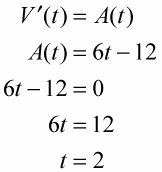Now, evaluate V(t) at the critical number, 2, and at the interval’s endpoints, 0 and 4: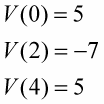So, the yo-yo has a maximum velocity of 5 inches per second twice — at both the beginning and the end of the interval. It reaches a minimum velocity of — 7 inches per second at t = 2 seconds.

## Total distance traveled

Total distance traveled is determined by adding up the distances traveled on each leg of the yo-yo’s trip: the up leg, the down leg, and the second up leg.

First, the yo-yo goes up from a height of 30 inches to about 31.1 inches (where the first turn-around point is). That’s a distance of about 1.1 inches. Next, it goes down from about 31.1 to about 16.9 (the height of the second turn-around point). That’s a distance of 31.1 minus 16.9, or about 14.2 inches. Finally, the yo-yo goes up again from about 16.9 inches to its final height of 18 inches. That’s another 1.1 inches. Add these three distances to obtain the total distance traveled: ~1.1 + ~14.2 + ~1.1 ≈ 16.4 inches.

Average speed is given by the total distance traveled divided by the elapsed time. Thus,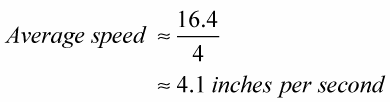## Maximum and minimum speed

You previously determined the yo-yo’s maximum velocity (5 inches per second) and its minimum velocity (-7 inches per second). A velocity of -7 is a speed of 7, so that’s the yo-yo’s maximum speed. Its minimum speed of zero occurs at the two turnaround points.

For a continuous velocity function, the minimum speed is zero whenever the maximum and minimum velocities are of opposite signs or when one of them is zero. When the maximum and minimum velocities are both positive or both negative, then the minimum speed is the lesser of the absolute values of the maximum and minimum velocities. In all cases, the maximum speed is the greater of the absolute values of the maximum and minimum velocities. Is that a mouthful or what?

## Maximum and minimum acceleration

Maximum and minimum acceleration may seem pointless when you can just look at the graph of A(t) and see that the minimum acceleration of –12 occurs at the far left when t = 0 and that the acceleration then goes up to its maximum of 12 at the far right when t = 4. But it’s not inconceivable that you’ll get one of those incredibly demanding calculus teachers who has the nerve to require that you actually do the math and show your work — so bite the bullet and do it.

To find the acceleration’s min and max from t = 0 to t = 4, set the derivative of A(t) equal to zero and solve: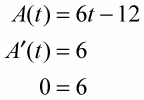This equation, of course, has no solutions, so there are no critical numbers and thus the absolute extrema must occur at the interval’s endpoints, 0 and 4.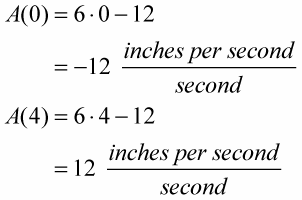Note that when the acceleration is negative — on the interval [0, 2) — that means that the velocity is decreasing. When the acceleration is positive — on the interval (2, 4] — the velocity is increasing.

## Speeding up and slowing down

Figuring out when the yo-yo is speeding up and slowing down is probably more interesting and descriptive of its motion than the info above. An object is speeding up (what we call “acceleration” in everyday speech) whenever the velocity and the calculus acceleration are both positive or both negative. And an object is slowing down (what we call “deceleration”) when the velocity and the calculus acceleration are of opposite signs.

Look at all three graphs in Figure 2 again. From t = 0 to about t = 0.47 (when the velocity is zero), the velocity is positive and the acceleration is negative, so the yo-yo is slowing town (until it reaches its maximum height).

When t = 0, the deceleration is greatest (12 inches per second per second; the graph shows an acceleration of negative 12, but here we’re calling it a deceleration so the 12 is positive). From about t = 0.47 to t = 2, both velocity and acceleration are negative, so the yo-yo is slowing down again (until it bottoms out at the lowest height).

Finally, from about t = 3.53 to t = 4, both velocity and acceleration are positive, so the yo-yo is speeding up again. The yo-yo reaches its greatest acceleration of 12 inches per second at t = 4 seconds.

## Tying it all together

Note the following connections among the three graphs in Figure 2. The negative section of the graph of A(t) – from t = 0 to t = 2 – corresponds to a decreasing section of the graph of V(t) and a concave down section of the graph H(t). The positive interval of the graph of A(t) – from t = 2 to t = 4 – corresponds to an increasing interval on the graph of V(t) and a concave up interval on the graph H(t). When t = 2 seconds, A(t) has a zero, V(t) has a local minimum, and H(t) has an inflection point.